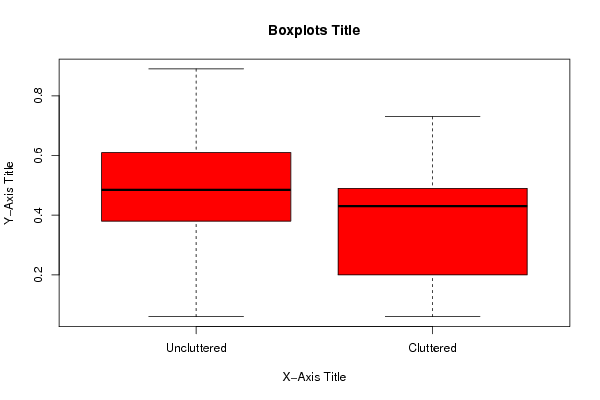## Free Statistics

of Irreproducible Research!

Author's title
Author*The author of this computation has been verified*
R Software Modulerwasp_Tests to Compare Two Means.wasp
Title produced by softwareT-Tests
Date of computationSat, 12 Nov 2011 20:50:53 -0500
Cite this page as followsStatistical Computations at FreeStatistics.org, Office for Research Development and Education, URL https://freestatistics.org/blog/index.php?v=date/2011/Nov/12/t1321149078r36u24t3g3kav4w.htm/, Retrieved Tue, 31 Jan 2023 00:41:35 +0000
Statistical Computations at FreeStatistics.org, Office for Research Development and Education, URL https://freestatistics.org/blog/index.php?pk=141542, Retrieved Tue, 31 Jan 2023 00:41:35 +0000
QR Codes:Original text written by user:
IsPrivate?No (this computation is public)
User-defined keywords
Estimated Impact82
Family? (F = Feedback message, R = changed R code, M = changed R Module, P = changed Parameters, D = changed Data)
-     [Aston University Statistical Software] [Morning Sickness ...] [2009-11-16 16:26:06] [74be16979710d4c4e7c6647856088456]
- R     [Aston University Statistical Software] [Morning Sickness ...] [2009-11-16 17:22:16] [74be16979710d4c4e7c6647856088456]
-   P     [T-Tests] [Morning Sickness ...] [2010-11-09 11:12:43] [3fdd735c61ad38cbc9b3393dc997cdb7]
- RM        [T-Tests] [Morning Sickness ...] [2011-11-07 09:34:35] [98fd0e87c3eb04e0cc2efde01dbafab6]
-             [T-Tests] [] [2011-11-13 01:41:50] [a2de6508349022a1eb68cf1e7e46129d]
-   PD          [T-Tests] [] [2011-11-13 01:44:32] [a2de6508349022a1eb68cf1e7e46129d]
-   PD              [T-Tests] [] [2011-11-13 01:50:53] [a24b35eb27a1a581879383db35460db1] [Current]
- R                   [T-Tests] [pattern] [2011-11-15 15:42:08] [b7df83e0fc4d05ddde076a5a0ec0675f]
Feedback Forum

Post a new message
Dataseries X:
0.06	0.24
0.12	0.73
0.61	0.53
0.89	0.44
0.59	0.45
0.31	0.07
0.67	0.28
0.6	0.45
0.23	0.73
0.44	0.41
0.6	0.67
0.21	0.45
0.66	0.06
0.39	0.33
0.78	0.42
0.57	0.41
0.38	0.52
0.59	0.49
0.48	0.55
0.44	0.13
0.3	0.45
0.63	0.19
0.43	0.2
0.74	0.07
0.48	0.11
0.77	0.45
0.37	0.4
0.61	0.45
0.49	0.19
0.46	0.51

 Summary of computational transaction Raw Input view raw input (R code) Raw Output view raw output of R engine Computing time 1 seconds R Server 'Herman Ole Andreas Wold' @ wold.wessa.net

\begin{tabular}{lllllllll}
\hline
Summary of computational transaction \tabularnewline
Raw Input & view raw input (R code)  \tabularnewline
Raw Output & view raw output of R engine  \tabularnewline
Computing time & 1 seconds \tabularnewline
R Server & 'Herman Ole Andreas Wold' @ wold.wessa.net \tabularnewline
\hline
\end{tabular}
%Source: https://freestatistics.org/blog/index.php?pk=141542&T=0

[TABLE]
[ROW][C]Summary of computational transaction[/C][/ROW]
[ROW][C]Raw Input[/C][C]view raw input (R code) [/C][/ROW]
[ROW][C]Raw Output[/C][C]view raw output of R engine [/C][/ROW]
[ROW][C]Computing time[/C][C]1 seconds[/C][/ROW]
[ROW][C]R Server[/C][C]'Herman Ole Andreas Wold' @ wold.wessa.net[/C][/ROW]
[/TABLE]
Source: https://freestatistics.org/blog/index.php?pk=141542&T=0

Globally Unique Identifier (entire table): ba.freestatistics.org/blog/index.php?pk=141542&T=0

As an alternative you can also use a QR Code:

The GUIDs for individual cells are displayed in the table below:

 Summary of computational transaction Raw Input view raw input (R code) Raw Output view raw output of R engine Computing time 1 seconds R Server 'Herman Ole Andreas Wold' @ wold.wessa.net

 T-Test Difference: Mean1 - Mean2 0.11733 T Statistic 2.19269 P-value 0.98175 Lower Confidence Limit -Inf Upper Confidence Limit 0.20826

\begin{tabular}{lllllllll}
\hline
T-Test \tabularnewline
Difference: Mean1 - Mean2 & 0.11733 \tabularnewline
T Statistic & 2.19269 \tabularnewline
P-value & 0.98175 \tabularnewline
Lower Confidence Limit & -Inf \tabularnewline
Upper Confidence Limit & 0.20826 \tabularnewline
\hline
\end{tabular}
%Source: https://freestatistics.org/blog/index.php?pk=141542&T=1

[TABLE]
[ROW][C]T-Test[/C][/ROW]
[ROW][C]Difference: Mean1 - Mean2[/C][C]0.11733[/C][/ROW]
[ROW][C]T Statistic[/C][C]2.19269[/C][/ROW]
[ROW][C]P-value[/C][C]0.98175[/C][/ROW]
[ROW][C]Lower Confidence Limit[/C][C]-Inf[/C][/ROW]
[ROW][C]Upper Confidence Limit[/C][C]0.20826[/C][/ROW]
[/TABLE]
Source: https://freestatistics.org/blog/index.php?pk=141542&T=1

Globally Unique Identifier (entire table): ba.freestatistics.org/blog/index.php?pk=141542&T=1

As an alternative you can also use a QR Code:

The GUIDs for individual cells are displayed in the table below:

 T-Test Difference: Mean1 - Mean2 0.11733 T Statistic 2.19269 P-value 0.98175 Lower Confidence Limit -Inf Upper Confidence Limit 0.20826

 Standard Deviations Variable 1 0.19709 Variable 2 0.1887

\begin{tabular}{lllllllll}
\hline
Standard Deviations \tabularnewline
Variable 1 & 0.19709 \tabularnewline
Variable 2 & 0.1887 \tabularnewline
\hline
\end{tabular}
%Source: https://freestatistics.org/blog/index.php?pk=141542&T=2

[TABLE]
[ROW][C]Standard Deviations[/C][/ROW]
[ROW][C]Variable 1[/C][C]0.19709[/C][/ROW]
[ROW][C]Variable 2[/C][C]0.1887[/C][/ROW]
[/TABLE]
Source: https://freestatistics.org/blog/index.php?pk=141542&T=2

Globally Unique Identifier (entire table): ba.freestatistics.org/blog/index.php?pk=141542&T=2

As an alternative you can also use a QR Code:

The GUIDs for individual cells are displayed in the table below:

 Standard Deviations Variable 1 0.19709 Variable 2 0.1887PNG link Postscript link PDF link

Parameters (Session):
par1 = less ; par2 = 1 ; par3 = 2 ; par4 = T-Test ; par5 = paired ; par6 = 0.0 ; par7 = 0.95 ; par8 = TRUE ;
Parameters (R input):
par1 = less ; par2 = 1 ; par3 = 2 ; par4 = T-Test ; par5 = paired ; par6 = 0.0 ; par7 = 0.95 ; par8 = TRUE ;
R code (references can be found in the software module):
par2 <- as.numeric(par2)par3 <- as.numeric(par3)par4 <- as.character(par4)par5 <- as.character(par5)par6 <- as.numeric(par6)par7 <- as.numeric(par7)par8 <- as.logical(par8)if ( par5 == 'unpaired') paired <- FALSE else paired <- TRUEx <- t(y)if(par8){bitmap(file='test1.png')(r<-boxplot(x ,xlab=xlab,ylab=ylab,main=main,notch=FALSE,col=2))dev.off()}load(file='createtable')if( par4 == 'Wilcoxon-Mann_Whitney'){a<-table.start()a <- table.row.start(a)a <- table.element(a,'Wilcoxon Test',3,TRUE)a <- table.row.end(a)a <- table.row.start(a)a <- table.element(a,'',1,TRUE)a <- table.element(a,'Statistic',1,TRUE)a <- table.element(a,'P-value',1,TRUE)a <- table.row.end(a)W <- wilcox.test(x[,par2],x[,par3],alternative=par1, paired = paired)a<-table.row.start(a)a<-table.element(a,'Wilcoxon Test',1,TRUE)a<-table.element(a,W$statistic[])a<-table.element(a,round(W$p.value, digits=5) )a<-table.row.end(a)a<-table.end(a)table.save(a,file='mytable.tab')}if( par4 == 'T-Test'){T <- t.test(x[,par2],x[,par3],alternative=par1, paired=paired, mu=par6, conf.level=par7)a<-table.start()a <- table.row.start(a)a <- table.element(a,'T-Test',3,TRUE)a <- table.row.end(a)if(paired){a <- table.row.start(a)a <- table.element(a,'Difference: Mean1 - Mean2',1,TRUE)a<-table.element(a,round(T$estimate, digits=5) )a <- table.row.end(a)}if(!paired){a <- table.row.start(a)a <- table.element(a,'Mean1',1,TRUE)a<-table.element(a,round(T$estimate, digits=5) )a <- table.row.end(a)a <- table.row.start(a)a <- table.element(a,'Mean2',1,TRUE)a<-table.element(a,round(T$estimate, digits=5) )a <- table.row.end(a)}a <- table.row.start(a)a <- table.element(a,'T Statistic',1,TRUE)a<-table.element(a,round(T$statistic, digits=5) )a <- table.row.end(a)a <- table.row.start(a)a <- table.element(a,'P-value',1,TRUE)a<-table.element(a,round(T$p.value, digits=5) )a <- table.row.end(a)a <- table.row.start(a)a <- table.element(a,'Lower Confidence Limit',1,TRUE)a<-table.element(a,round(T$conf.int, digits=5) )a <- table.row.end(a)a<-table.row.start(a)a <- table.element(a,'Upper Confidence Limit',1,TRUE)a<-table.element(a,round(T\$conf.int, digits=5) )a <- table.row.end(a)a<-table.end(a)table.save(a,file='mytable.tab')}a<-table.start()a <- table.row.start(a)a <- table.element(a,'Standard Deviations',3,TRUE)a <- table.row.end(a)a <- table.row.start(a)a <- table.element(a,'Variable 1',1,TRUE)a<-table.element(a,round(sd(x[,par2]), digits=5) )a <- table.row.end(a)a <- table.row.start(a)a <- table.element(a,'Variable 2',1,TRUE)a<-table.element(a,round(sd(x[,par3]), digits=5) )a <- table.row.end(a)a<-table.end(a)table.save(a,file='mytable1.tab')